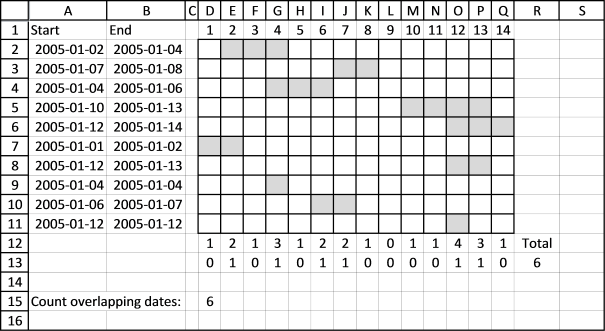Author: Oscar Cronquist Article last updated on October 09, 2018

In the previous post I explained how to count overlapping dates between a single date range and multiple date ranges. In this post I will demonstrate how to count overlapping dates among multiple date ranges.The date ranges are in column A and B. The chart to the right is there so that you can easily verify the formula.

### Count the number of overlapping date ranges for each date

The first formula returns an array that counts the number of overlapping date ranges for each date. It is shown in cell range D12:Q12.

=MMULT(TRANSPOSE(\$A\$2:\$A\$11^0), (TRANSPOSE(MIN(\$A\$2:\$A\$11)+ROW(A1:INDEX(\$A:\$A, MAX(\$B\$2:\$B\$11)-MIN(\$A\$2:\$A\$11)+1))-1)>=\$A\$2:\$A\$11)*((TRANSPOSE(MIN(\$A\$2:\$A\$11)+ROW(A1:INDEX(\$A:\$A, MAX(\$B\$2:\$B\$11)-MIN(\$A\$2:\$A\$11)+1))-1))<=\$B\$2:\$B\$11))

The formula returns this array: {1, 2, 1, 3, 1, 2, 2, 1, 0, 1, 1, 4, 3, 1}

Date 2005-01-01 is overlapped once by date range 2005-01-01/2005-01-02. So the first value in the array is 1.
Date 2005-01-02 is overlapped twice by date range 2005-01-01/2005-01-02 and 2005-01-02/2005-01-04. The second value in the array is 2.
...
Date 2005-01-09 is not overlapped at all so the ninth value in the array is 0 (zero).
And so on.., verify these numbers with the chart above.

### Count dates overlapped by two or more date ranges

The second array formula returns an array that indicates if a date is overlapped by two or more date ranges. It is entered in cell range D13:Q13.

=(MMULT(TRANSPOSE(\$A\$2:\$A\$11^0), (TRANSPOSE(MIN(\$A\$2:\$A\$11)+ROW(A1:INDEX(\$A:\$A, MAX(\$B\$2:\$B\$11)-MIN(\$A\$2:\$A\$11)+1))-1)>=\$A\$2:\$A\$11)*((TRANSPOSE(MIN(\$A\$2:\$A\$11)+ROW(A1:INDEX(\$A:\$A, MAX(\$B\$2:\$B\$11)-MIN(\$A\$2:\$A\$11)+1))-1))<=\$B\$2:\$B\$11))>1)*1

This formula returns {0, 1, 0, 1, 0, 1, 1, 0, 0, 0, 0, 1, 1, 0}

If we sum this array we get the total number of overlapped dates. That value is shown cell D15 and R13.

Functions in this post: MMULT, ROW, INDEX

Interested in learning more about excel formulas? Join my Advanced excel course.